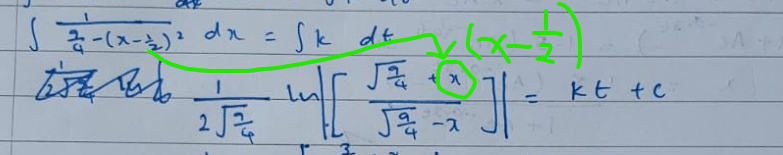# Gayathri

### Transformations worksheet

Transformations_August

### Plan for 28th June

• Recommended questions: CJC 2018 MYE
• Extra (optional). Q11 is hypothesis testing so we can skip that for now MJC 2018 MYE

Paper 1

• Q1,2: Good!
• Q3: Look for the line right after the “shown” line in your working. In the by parts step, when we differentiate the “$u^2$”, we should have “$2u$” so we’re missing a “2” in our working. All other steps look good.
• Q4(i). Add in these two key words: Since every horizontal line $y=k$ cuts the graph of $y=f(x)$ at most once, …
• Q4(iii). For show questions good to give a bit more details. Your line $R_f \not \subseteq D_f$ is good, but even better if we start off by copying down what $R_f$ and $D_f$ is.
• Q5(ii) For qns that ask us for reason, good to give a short word statement: “Hence $\displaystyle \sum_{r=1}^\infty \frac{1}{4r^2-1}$ converges” after the $\frac{1}{4n+2}\to 0$ line.
• Q6(iii) Your answer is good: keep it up! If you want the most simplified answer, whenever we see a “big” power on our surds, we can further simplify it by taking out all even powers. For example $(\sqrt{3})^5 = (\sqrt{3})^4 \sqrt{3} = 9 \sqrt{3}$. So for our coordinates of $A$, we can further simplify $(\sqrt{\frac{2}{3}})^3$ into $\frac{2}{3}\sqrt{\frac{2}{3}}$.
A similar approach can be used for Q7(ii) to simplify $(\sqrt{8})^7$ into $1024 \sqrt{2}$.
• Q9(iii) Careless at the last step. $\left | \begin{pmatrix} 6 \\ 2 \\ 4 \end{pmatrix} \right |$ is $\sqrt{56}$, not 56.
• Q10(a)(ii) Careless in the differentiation: the 13 should disappear after differentiation.

Paper 2

• Q1(a): For $y=3f(x)$, we are stretching parallel to the $y$-axis by factor $3$ (rather than $\frac{1}{3}$ for $x$-axis stretching like $y=f(3x)$). So final $y$-coordinate should be $3b$ rather than $\frac{b}{3}$.
• Q2(ii): Look for the line $an=3$ in your working. That step is correct. Careless in the next step where we had $a = \frac{n}{3}$ vs the correct step of $a = \frac{3}{n}$.
• Q4(ii):• Q8(vi) Your answer is good! The short, mathematical way to phrase that will be that the event in part (iv) is a proper subset of the event in part (v).
• Q8(vii) You might want to double check your GC/table working. The answer should be 8 rather than 7 (all above steps look good).
• Q9(ii) Almost there. There’s an extra step of choosing which boys and girls to sit down. So we must further multiply by $\binom{5}{2} \times \binom{6}{3}$ to get the actual answer.
• Q9(iv): need an extra $\times 2!$ to account for the fact that either guys or girls could be first.

### TPJC Paper: Things to discuss together during class

Paper 1

• Q5(iii): use of “sum” vs “term” after the substitution step.
• Q7(iii): getting the 3 smallest positive integers for $n$ (using trial and error and the GC).
• Q8(iv)
• Q9(iii): Hence method
• Q10(a)(iii) Sketching
• Q10(b)

Paper 2

• Q4(iii) Recommendation in dealing with the modulus in DE questions.
• Q6(ii)
• Q9(iii)(a)
• Q10 (iii)

### 21st-28th May HW discussion

• Summation: CJC (i), (iii) (don’t need to redo MOD), DHS (replacement method), Q2(ii), NYJC (can use GC for 3), SAJC (replacement method)
• 2015 TYS: P1 Q10 (careless), Q11(iv), P2 Q3a (add $k\in\mathbb{R}$ and change ‘only’ to ‘at most’), Q4b (Set notation), Q4b (sign change when ‘flipping’), Q11(iv).

### 14th-21st May HW discussion

• To look at on your own
• Binomial Q9a) Just key the last line in your calculator again. Should get the answer behind. Working looks good.
• 2015 TYS: Q1: In your working you had this line: $-2.4 = \frac{a}{1.6^2} + b(1.6) + c$. To get to the next line, I think your multiplied throughout by $1.6^2$. But in doing that, every term must be multiplied by $1.6^2$, including the guys behind with $b$ and $c$. So the correct equation (1) should be $-6.144 = a + 1.6^3 b + 1.6^2 c$.
Equation (2) the same mistake was made.
The rest of the question (equation (3)) and later parts) are all correct from ecf.
• Q4: To get full marks for this question we’d also need to prove the the area we obtained is indeed a maximum.
• To discuss during class later
• Binomial Q7bi, 9b,d
• 2015 TYS: Q3, Q5(ii),(iii), Q7(iii), Q11 (set notation)

### Plan for 14th May

• Review questions from school/HW
• DRV Q5b, 7, binomial presentation, binomial basic questions q1(ii), 4(iii),(iv), 5(i),(iii)
• Past prelim questions (tables, graphs, combinations with other topics, mode): Q1-4: S4_Binomial_2020
• Start on revision of earlier topics/other papers
• TYS P1 Q1-5 2015

### HW for 14th-21st May

• Binomial Past Prelim Questions (S4) Q6a,b, 8, 9

### HW for 7th-14th May

• TBD (DRV/Binomial/Past topics/Past papers).

### Tentative plan for 30th April

• Review any questions from last week/school/tutorial
• DRV Q2-6, Q10: S3_DRV
• If time permits, start discussion on binomial
• Binomial Basic Questions
• Discuss holiday revision plan

### Tentative plan for 16th April:

• Review any questions from last week/school/tutorial
• P&C Worksheet Q12b: Using Venn diagrams
• Continue with Probability work: discussions on conditional probability, independent events and mutually exclusive events. (we will do Q1 and Q2 below together)
• Continue on this worksheet: Probability Basic Questions
• P&C Worksheet Q14c: Use of conditional probability
• Start drill work on probability (past prelim questions): Probability Worksheet

### Tentative plan for 9th April:

• Review any questions from last week/school/tutorial
• Continue with P&C work: Q11-14
• Start discussion on probability: Probability Summary Notes
• Look at some probability questions together: Probability Basic Questions
• Tree diagram: Q1
• Venn diagram: Q3
• Independent events: Q2